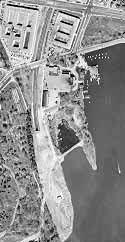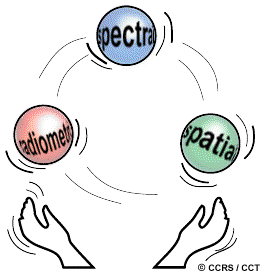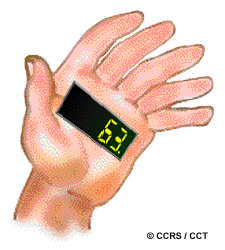## Search

While the arrangement of pixels describes the spatial structure of an image, the radiometric characteristics describe the actual information content in an image. Every time an image is acquired on film or by a sensor, its sensitivity to the magnitude of the electromagnetic energy determines the radiometric resolution. The radiometric resolution of an imaging system describes its ability to discriminate very slight differences in energy The finer the radiometric resolution of a sensor, the more sensitive it is to detecting small differences in reflected or emitted energy.Figure 1 - Text Version

## Figure 1

These two images show a port area. The images are in grey scale. The illustration on the left shows the image presented in two bits, or 4 shades of grey. The illustration on the right is the same but presented in 8 bits or 256 shades of grey, which provides more details. This collage shows the difference in the level of detail between the two representations.

Imagery data are represented by positive digital numbers which vary from 0 to (one less than) a selected power of 2. This range corresponds to the number of bits used for coding numbers in binary format. Each bit records an exponent of power 2 (e.g. 1 bit=2 1=2). The maximum number of brightness levels available depends on the number of bits used in representing the energy recorded. Thus, if a sensor used 8 bits to record the data, there would be 28=256 digital values available, ranging from 0 to 255. However, if only 4 bits were used, then only 24=16 values ranging from 0 to 15 would be available. Thus, the radiometric resolution would be much less. Image data are generally displayed in a range of grey tones, with black representing a digital number of 0 and white representing the maximum value (for example, 255 in 8-bit data). By comparing a 2-bit image with an 8-bit image, we can see that there is a large difference in the level of detail discernible depending on their radiometric resolutions.

## Did you know?

"...you just can't have it all!..."Figure 2 - Text Version

## Figure 2

This illustration shows two hands juggle three balls representing radiometric, spectral and spatial resolution. This demonstrates that the user must continuously make tradeoffs between these three resolutions.

...that there are trade-offs between spatial, spectral, and radiometric resolution which must be taken into consideration when engineers design a sensor. For high spatial resolution, the sensor has to have a small IFOV (Instantaneous Field of View). However, this reduces the amount of energy that can be detected as the area of the ground resolution cell within the IFOV becomes smaller. This leads to reduced radiometric resolution - the ability to detect fine energy differences. To increase the amount of energy detected (and thus, the radiometric resolution) without reducing spatial resolution, we would have to broaden the wavelength range detected for a particular channel or band. Unfortunately, this would reduce the spectral resolution of the sensor. Conversely, coarser spatial resolution would allow improved radiometric and/or spectral resolution. Thus, these three types of resolution must be balanced against the desired capabilities and objectives of the sensor.

## Whiz quizFigure 3 - Text Version

## Figure 3

This illustration shows the two hands of a person trying to count on his fingers to get the answer to the quiz question.

Suppose you have a digital image which has a radiometric resolution of 6 bits. What is the maximum value of the digital number which could be represented in that image?Figure 4 - Text Version

## Figure 4

This illustration shows the hand of a person with the number 63 written on it.

The number of digital values possible in an image is equal to the number two (2 - for binary codings in a computer) raised to the exponent of the number of bits in the image (i.e. 2# of bits). The number of values in a 6-bit image would be equal to 26 = 2 x 2 x 2 x 2 x 2 x 2 = 64. Since the range of values displayed in a digital image normally starts at zero (0), in order to have 64 values, the maximum value possible would be 63.